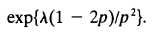### Create an Account

Home / Questions / In a branching process the number of offspring per individual has a binomial distribution ...

# In a branching process the number of offspring per individual has a binomial distribution with parameters 2 p Starting with a single individual calculate a the extinction probability

In a branching process the number of offspring per individual has a binomial distribution with parameters 2,p. Starting with a single individual, calculate:

(a) the extinction probability;

(b) the probability that the population becomes extinct for the first time in the third generation

Suppose that, instead of starting with a single individual, the initial population size 4 is a random variable that is Poisson distributed with mean A. Show that, in this case, the extinction probability is given, for p > 1/2, byAug 02 2020 View more View LessSubscribe To Get Solution